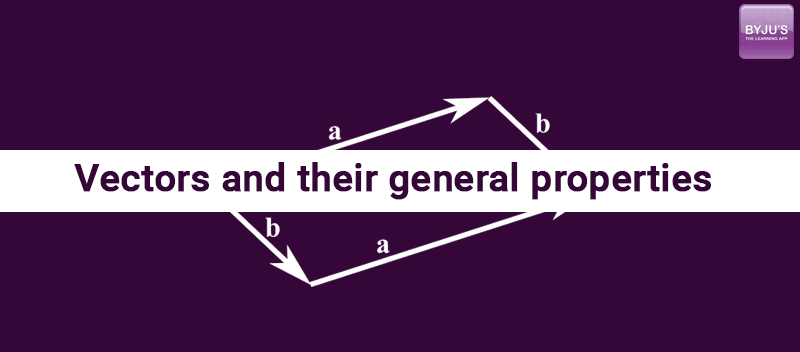Jet Set Go! All about Aeroplanes Jet Set Go! All about Aeroplanes

# Vectors and Their General PropertiesWe already learned about the vectors and their notations in the previous article. Now we will learn about the general properties of vectors, and we will see what a unit vector is.

### Equality of Vectors:

When we are talking about the equality of two vectors, they must represent the same physical quantity. Two vectors are said to be equal if their magnitudes and directions are the same. Therefore, we can conclude that a parallel translation of a vector does not bring about any change in it.

### Multiplication of a vector by a Number:

Suppose
a is a vector of magnitude ‘a’, and n is a number. If we multiply a by n, then we will get a new vector. Let’s say this vector is b

Vector b = nx vector a

The magnitude of vector b = na
The direction of vector b = Direction of vector a
(If n = positive value)

The direction will be reversed if “n” will have a negative value

Note: if vector b is opposite to vector a, then n = -1

### Unit vector:

A unit vector is a vector of magnitude (or length) of 1 unit. Thus, unit vectors are used to specify the directions of vector quantities in various coordinate systems. In Cartesian coordinates, generally:

$$\begin{array}{l} \hat {i} \textup{= unit vector in x-direction} \end{array}$$

$$\begin{array}{l} \hat {j} \textup{= unit vector in y-direction} \end{array}$$
$$\begin{array}{l} \hat {k} \textup{= unit vector in z direction} \end{array}$$

The position vector of any object can be represented in Cartesian coordinates as:

$$\begin{array}{l} \overrightarrow {r} = x \hat{i}~+~ y \hat{j}~+~ x \hat{k} \end{array}$$

There is a lot more to know about vectors. Knowing about vectors is the first and basic step towards learning higher physics as it deals with a lot of vector quantities. Stay tuned with BYJU’S to learn more about vectors, vectors notations much more.

Test your Knowledge on Properties Of Vector